# A Self-Descriptive Crossword Puzzle

From Lee Sallows:

Can you complete the ‘self-descriptive crossword puzzle’ at left below? As in the solution to a similar puzzle seen at right, each of its 13 entries, 6 horizontal, 7 vertical, consists of an English number name folowed by a space followed by a distinct letter. The number preceding each letter describes the total number of occurrences of the letter in the completed puzzle. Hence, in the example, E occurs thirteen times, G only once, and so on, as readers can check. Note that the self-description is complete; every distinct letter is counted.

Though far from easy, the self-descriptive property of the crossword enables its solution to be inferred from its empty grid using reasoning based on orthography only.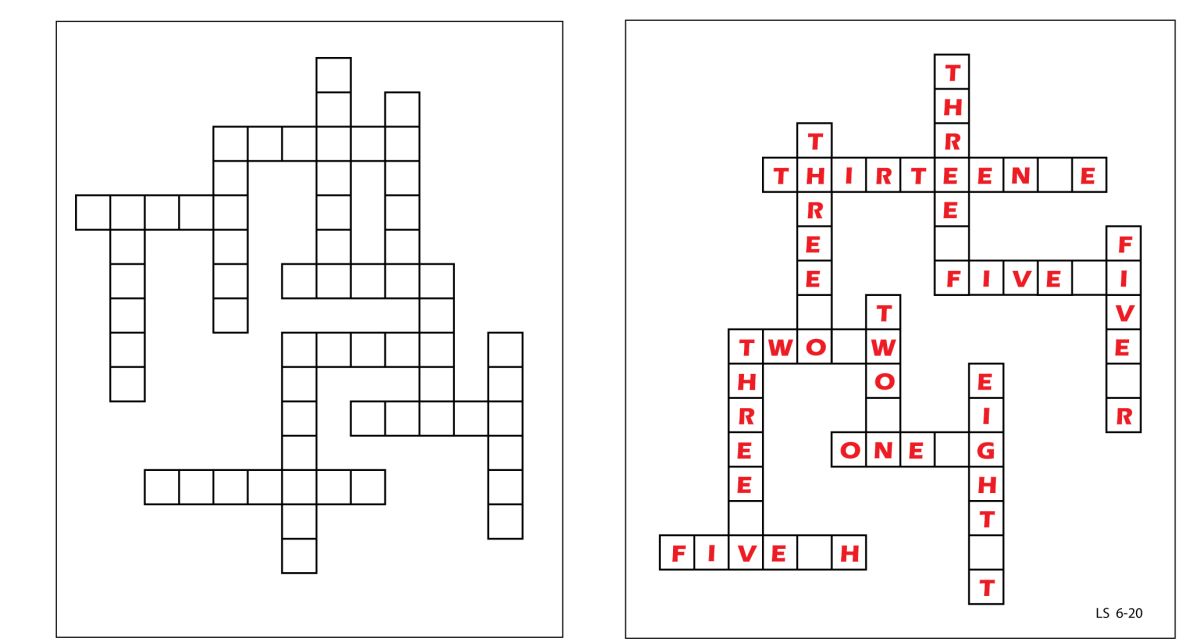# Reciprocation

Another remarkable contribution by Lee Sallows: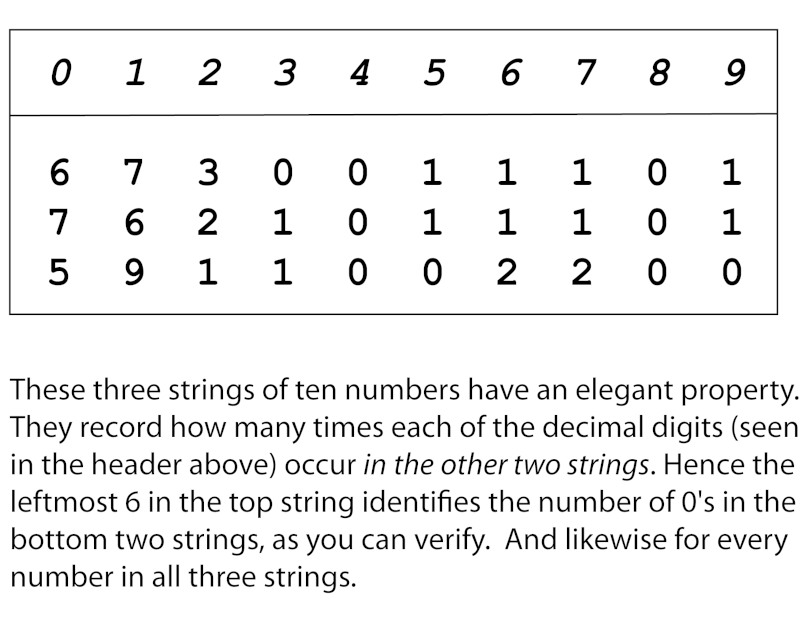(Thanks, Lee!)

# A Panmagic Geomagic Square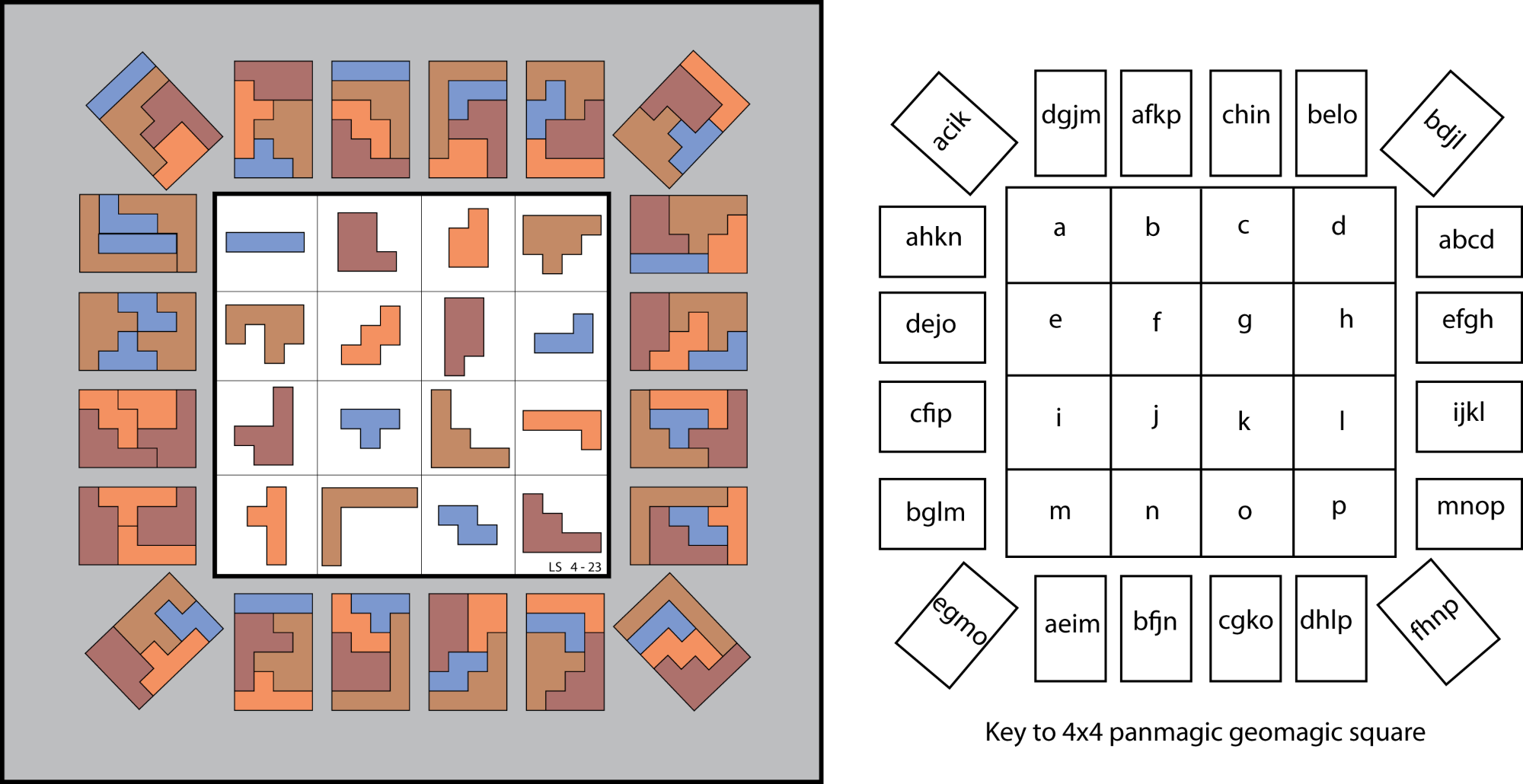Another amazing contribution by Lee Sallows:

“The picture above shows a 4×4 geomagic square, which is to say a magic square using geometrical shapes that can be fitted together so as to form an identical target shape, in this case a 4×6 rectangle, rather than numbers adding to a constant sum. In addition, the square is also panmagic, meaning that besides the usual 4 rows, 4 columns, and both main diagonals, the shapes occupying each of the so-called ‘broken’ diagonals, afkn, dejo, cfip, bglm, chin, belo, are also able to tile the rectangle. Lastly, the 4 shapes contained in the corner cells of the four embedded 3×3 sub-squares, acik, bdjl, fhnp, egmo, are also ‘magic’, bringing the total number of target-tiling shape sets to 20, a small improvement over the 16 achieved by a panmagic-only square. With that said, it is worth noting that 4×4 geomagic squares have been found achieving target-tiling scores as high as 48.”

Click the image to expand it. Thanks, Lee!

From Lee Sallows:

Earlier this year, Futility Closet featured a puzzle based upon the well-known 7-segment display. Less well known is the 15-cell display shown in Figure 1, in which each decimal digit appears as a pattern of highlighted cells within a 3×5 rectangle. Call these the small rectangles. Observe also that the digit 1 is represented as the vertical column of 5 cells in the centre of its small rectangle rather than as either of the two alternative columns immediately to left and right, a detail that is important in view of what follows.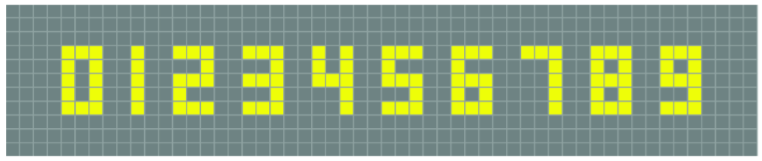Figure 1.

Figure 2 shows a pair of readouts using 15-cell displays each arranged in the form of a (large) 3×5 rectangle that mirrors the smaller rectangles just mentioned. The two readouts describe each other. The top left cell in the right-hand readout contains the number 18. A check will show that the number of highlighted top left cells appearing in the left-hand readout is indeed 18. Take for instance the top left-hand cell in the left-hand readout. It contains the number 17, which employs two digits, 1 and 7. None of the 5 cells forming the digit 1 is in top left position within its small rectangle. But the leftmost cell in digit 7 is indeed in top left position. Proceeding next to the left-hand readout’s top centre cell we find two cells in top left position: one in digit 2 and one in digit 4. The score of top left cells so far is thus 1+2 = 3. Continuing in normal reading order, a list of the left-hand readout numbers followed by their top left cell scores in brackets is as follows: 17 (1), 24 (2), 17(1), 13(1), 9(1), 15(1), 17(1), 25(2), 17(1), 8(1), 9(1), 14(1), 15(1), 24(2), 17(1). The sum of the scores is 18, as predicted.

In the same way, a number occupying position x in either of the readouts will be found to identify the total number of cells occurring in position x within the digits of the other readout. That is, the two readouts are co-descriptive, they describe each other.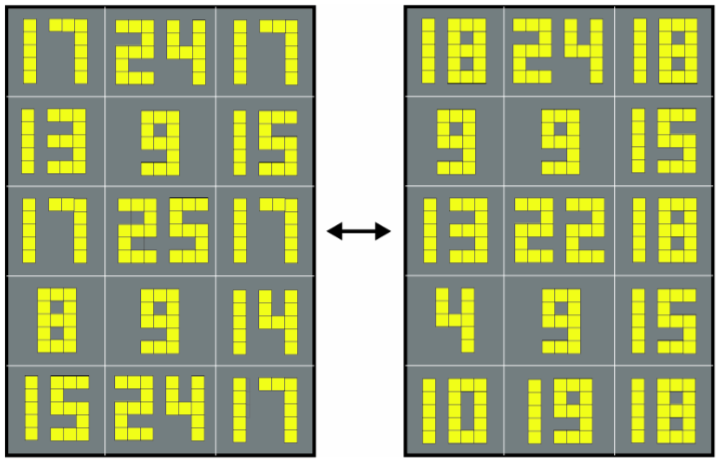Figure 2.

Recalling now the solution to the earlier mentioned 7-segment display puzzle, some readers may recall that it involved an iterative process that terminated in a loop of length 4. Likewise, the pair of readouts in Figure 2 are the result of a similar process, but now terminating in a loop of length 2. In that case we were counting segments, here we are counting cells. An obvious question thus prompted is: What kind of a readout would result from a loop of length 1? The answer is simple: a description of the readout resulting from a loop of length 1 would be a copy of the same readout. That is, it will be a self-descriptive readout, the description of which is identical to itself. Such a readout does indeed exist. Can the reader find it?

# “Extra Magic” Realized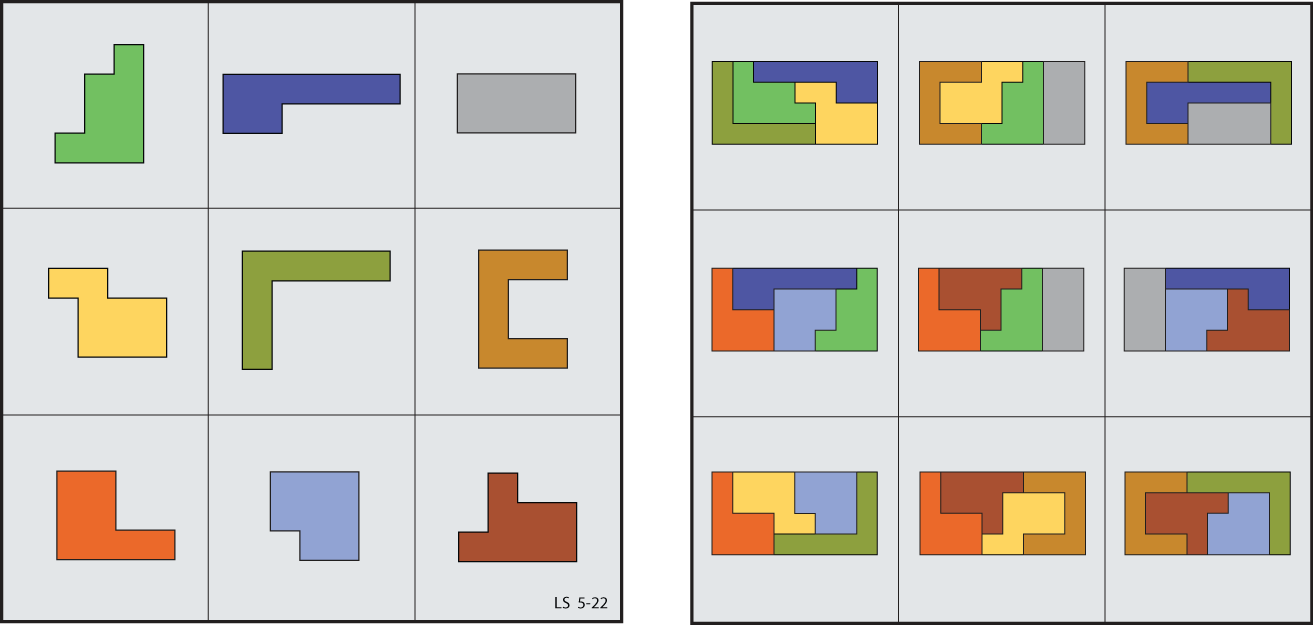From Lee Sallows:

The drawing at left above shows an unusual type of 3×3 geomagic square, being one in which the set of four pieces occupying each of the square’s nine 2×2 subsquares can be assembled so as to tile a 4×8 rectangle. The full set of subsquares become more apparent when it is understood that the square is to be viewed as if drawn on a torus, in which case its left-hand and right-hand edges will coincide, as will its upper and lower edges. In an earlier attempt at producing such a square several of the the pieces used were disjoint, or broken into separated fragments. Here, however, the pieces used are nine intact octominoes.

(Thanks, Lee!)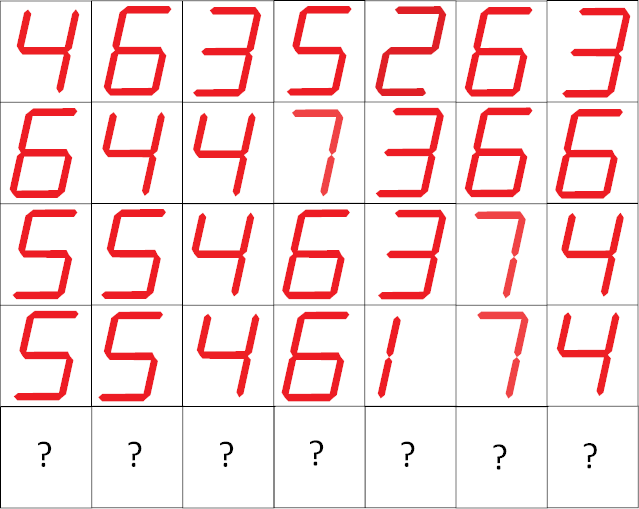A puzzle by Lee Sallows. In this readout from a computer-driven electronic display, the digits in the fifth row have been obscured. What are they?

# Extra-ordinary Magic

From Lee Sallows:

A recent contribution to Futility Closet showed an atypical type of 3×3 geometric magic square in which the 4 pieces occupying each of its nine 2×2 subsquares are able to tile the same rectangle. A different square with the same property is seen in the figure here shown, where the nine tiled rectangles appear at right.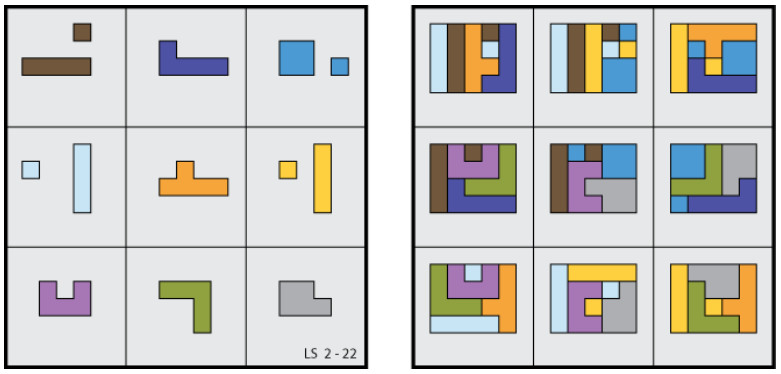As in the earlier example, the square is to be interpreted as if drawn on a torus, the relations among its peripheral cells then being the same as those that result if the square is surrounded with copies of itself, as seen in the following figure showing four such copies, one in each quadrant: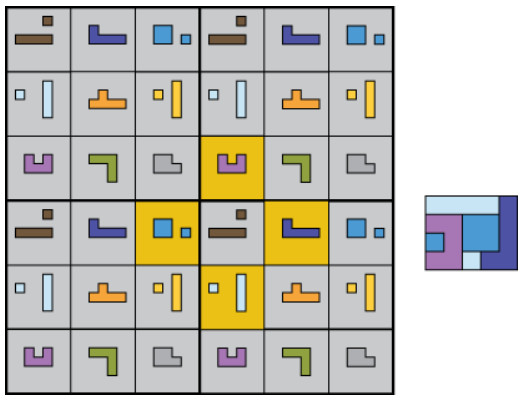The figure makes it easier to identify the different 2 × 2 subsquares, exactly nine distinct examples of which can be identified. A brief commentary on the square pointed out that the number of ‘magic’ conditions it satisfies is one greater than the eight conditions demanded by a conventional 3 × 3 magic square. Hence the title of the piece, ‘Extra Magic.’

It was while perusing this diagram that an alternative division of the cells into sets of 4 suggested itself. Instead of 2 × 2 subsquares, consider the four cells defined by a cross that can be centered on any chosen cell. The above figure shows a yellow-shaded example, along with a rectangle tiled by its four associated shapes. It is interesting to note that, as before, there are just nine distinct crosses of this kind to be found in a 3 × 3 square. An obvious question thereby prompted was whether or not a new 3 × 3 magic square could be found based upon such crosses rather than 2×2 subsquares? The answer turned out to be yes, but in the process of scrutinizing an initial specimen I noticed that although it embodied nine cross-based sets of 4 rectangle-tiling pieces, as required, it also included a couple of additional rectangle-tiling sets contained within 2 × 2 subsquares. Clearly the maximum number of such surplus sets would be nine, one for each cross, but could a specimen showing nine cross-based and nine subsquare-based rectangle-tiling sets really exist? I lost no time in seeking an answer.

Regrettably, I was unable to find one. However, the figure below shows a close approach to perfection. It is the same 3 × 3 square with which we started, but now shown alongside no less than eight additional rectangles, each of them tiled with a set of 4 pieces belonging to a cross. Note that the missing rectangle is the one belonging to the non-magic central cross, a show of symmetry that seems appropriate.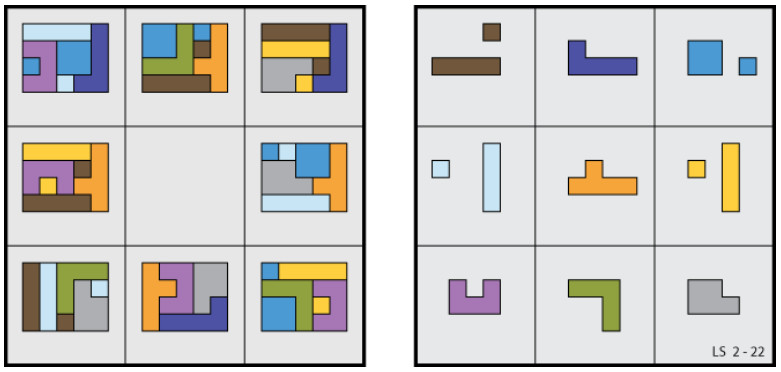So whereas a 3 × 3 magic square, numerical or geometric, satisfies at least 8 separate conditions (3 rows + 3 columns + 2 diagonals), the square here shown satisfies no less than eight more.

(Thanks, Lee.)

# Extra Magic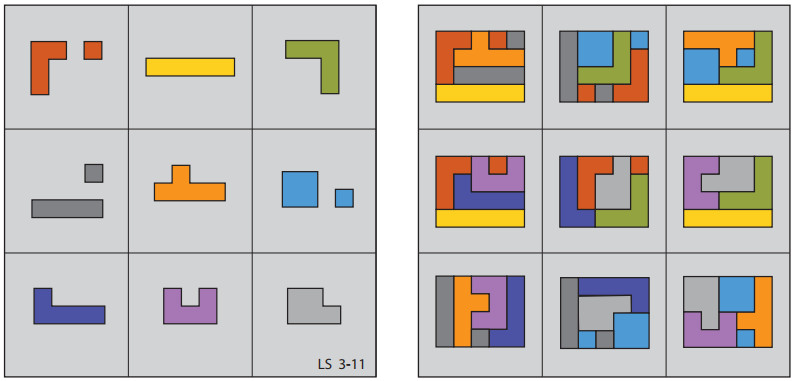From Lee Sallows:

The traditional magic square is a square array of n×n distinct numbers, their magical property being that the sum of the n numbers occupying each row, column, and diagonal is the same. A variation on this theme that I introduced in 2011 is the geometric magic square in which distinct geometrical figures (usually planar shapes) occupy the cells of the array rather than numbers. The magical property enjoyed by such an array is then that the n shapes making up each row, column, and diagonal can be fitted together as in a jigsaw puzzle so as to yield (i.e. tile) a new compound shape that is the same in each case.

Beyond ‘ordinary’ geometric magic squares, it turns out that the combinative properties of shapes are such as to enable ‘magical’ constructions that are denied to analogous structures using numbers. For example, at left in the figure above is seen a 3×3 square of a kind that cannot be realized using distinct numbers rather than shapes. Note first that the square is to be understood as ‘toroidally-connected’, which is to say, as if inscribed on a torus. Its left-hand edge is then to be interpreted as adjacent to its right-hand edge and its top edge adjacent to its bottom edge. Its magical property is then that the four pieces contained within any 2×2 subsquare can be assembled to produce an identical shape, in this case a rectangle of size 4×5. In all there are nine such subsquares to be found in the square, as seen (again in a square) at right. Note that three of the pieces are disjoint, my attempts to produce a similar solution using nine unbroken pieces having failed. So whereas a 3×3 magic square, numerical or geometric, satisfies at least 8 separate conditions ( 3 rows + 3 columns + 2 diagonals), the square here shown satisfies one more.

(Thanks, Lee!)